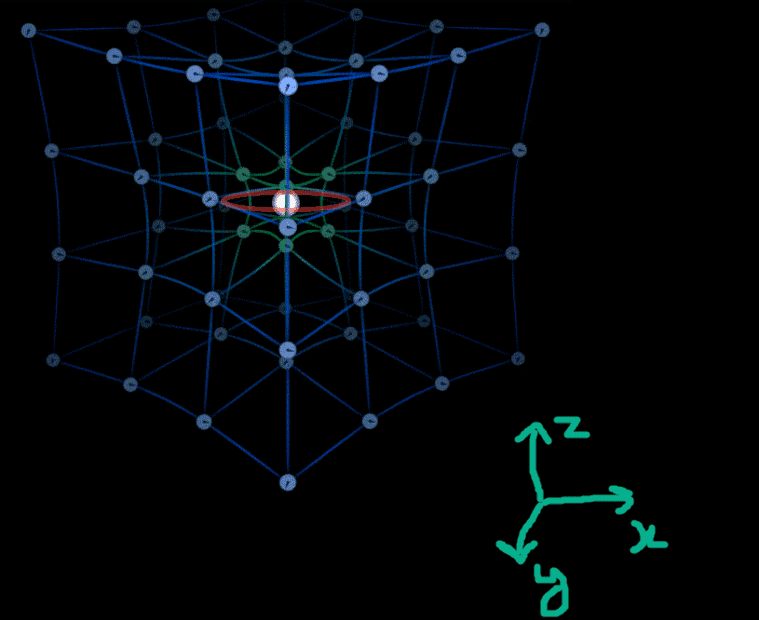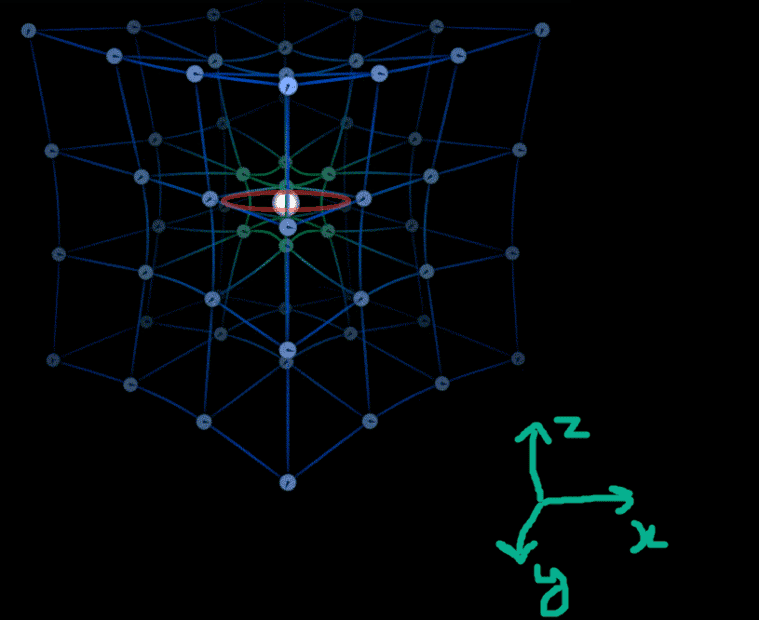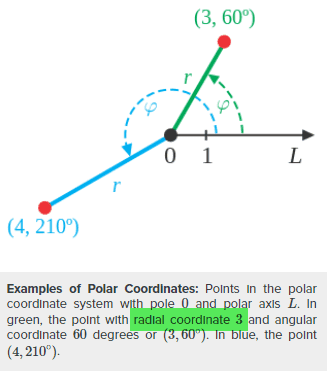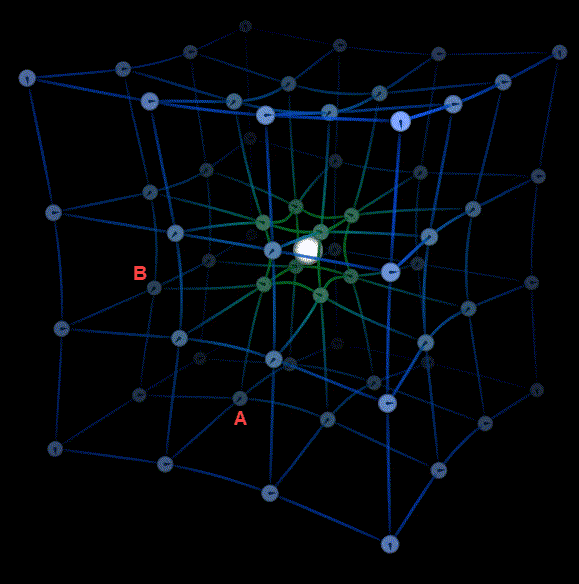# Moving along one axis in a four-dimensional plane

• B
PainterGuy
TL;DR Summary
If one is moving along only one dimension, such as x-axis, in four-dimensional plane, are the coordinates of other axes, y, z, w, going to remain constant? "w" is the fourth axis.
Hi,

When you have a one-dimensional plane, such as x-axis plane, when you move, you coordinates will change along all given axes. Actually, there is only one dimension available in this case so it doesn't make much sense here.

When you have two-dimensional plane, such as x-y plane, when you move, you can keep coordinate of at most one dimension constant. For example, if you decide to move along x-axis, your coordinate for y-axis won't change.

When you have a three-dimensional plane, such as x-y-z plane, when you move, you have the freedom of keeping the coordinates of at most two dimensions same. For example, if you move only along x-axis, your coordinates for y- and z-axis won't change.

Now suppose you have a four-dimensional plane, the coordinates of how many dimensions could be kept constant, at most, while moving along one dimension such as x-axis? Three dimensions? Could you please help me with this?

Mentor
It depends. As a purely geometric view, one can easily imagine keeping all coordinates the same while changing just one and essentially mapping out a line in space — think 3D, 4D …n-dimensional.

However if you’re thinking about spacetime and the real world then time is always changing whether x,y,z changes or not.

•PainterGuy
Mentor
A plane is by definition two-dimensional, so it doesn't make sense to talk about a three-dimensional plane or one of higher dimensions. We can embed a (two-dimensional) plane in three-dimensional space, and we can embed a three-dimensional hyperplane in four dimensions. A hyperplane (see https://en.wikipedia.org/wiki/Hyper..., its hyperplanes are the 1-dimensional lines.) will always be of one dimension less than the space it's embedded in.

Aside from four-dimensional spacetime as already mentioned, if you have a 3D hyperplane embedded in a Euclidean 4D space, if an object travels along one axis, all of the other coordinates will not change.

•PainterGuy
PainterGuy
Since the space-time was mentioned, I thought it's better to clarify a related point.

I have seen the following image at different places. The image tries to incorporate time dimension in regular 3D space. If the mass is spherically symmetric then it'd distort the time around it. The time on the clocks, lying along the circle equidistant from the mass, would be affected by equal amount. In the image below the red circle lies in x-y plane and white sphere represents mass.

If one moves along the red circle, this way time and z-axis coordinate won't change. It it okay to think along these lines? Could you please guide me?Source: https://steemitimages.com/p/3zpz8WQ...dYyDNYDRhZDjxXE6um9eWTf?format=match&mode=fit

Homework Helper
Gold Member
2022 Award
I have seen the following image at different places. The image tries to incorporate time dimension in regular 3D space.
You can only really show time in addition to one or two spatial dimensions, but not three in the same diagram. Unless you repeat the diagram as it changes over time - like in a movie!
If the mass is spherically symmetric then it'd distort the time around it. The time on the clocks, lying along the circle equidistant from the mass, would be affected by equal amount. In the image below the red circle lies in x-y plane and white sphere represents mass.
That's just a circle in space (in this referece frame).
If one moves along the red circle, this way time and z-axis coordinate won't change. It it okay to think along these lines?
Motion is the change in spatial position over time, so talking about "moving" while keeping time constant is not physically very meaningful. If time is not changing, then you have a spatial structure of some description - like a circle in a plane.

•hutchphd and PainterGuy
PainterGuy
Thank you for the help!

You can only really show time in addition to one or two spatial dimensions, but not three in the same diagram. Unless you repeat the diagram as it changes over time - like in a movie!

If you look the original diagram from my previous post, it shows changing time clocks. I think it's closer to what you are suggesting.

Motion is the change in spatial position over time, so talking about "moving" while keeping time constant is not physically very meaningful. If time is not changing, then you have a spatial structure of some description - like a circle in a plane.

I think my choice of words was not good. I was trying to say that all the clocks lying around that circle measure the flow of time at the same rate so if a person is moving around that circle, "rate of flow of time" and the z-axis coordinate would remain the same. Yes, the time itself would change because it would need some time to move from one point on the circle to another point. Does it make any sense now? Thanks for the help, in advance!

For your convenience, I'm attaching the diagram from my previous post again.Source: https://steemitimages.com/p/3zpz8WQ...dYyDNYDRhZDjxXE6um9eWTf?format=match&mode=fit

Homework Helper
Gold Member
2022 Award
I think my choice of words was not good. I was trying to say that all the clocks lying around that circle measure the flow of time at the same rate so if a person is moving around that circle, "rate of flow of time" and the z-axis coordinate would remain the same. Yes, the time itself would change because it would need some time to move from one point on the circle to another point. Does it make any sense now? Thanks for the help, in advance!
What specifically are you trying to understand from that?

"Rate of flow of time" is an unusual way to describe it. Gravitational time dilation is the more usual terminology. Clocks at different radial coordinates show time dilation relative to each other.

•PainterGuy
PainterGuy
What specifically are you trying to understand from that?
I'm more of curious.

Clocks at different radial coordinates show time dilation relative to each other.

In the diagram below as you move around in a circle, your radial coordinate won't change but your angular coordinate would do. So, in the diagram from my previous post, as you move around in a circle your radial coordinate won't change and hence the clocks won't show any time dilation relative to each other. Could you please confirm this?Source: https://courses.lumenlearning.com/boundless-algebra/chapter/the-polar-coordinate-system

Homework Helper
Gold Member
2022 Award
In the diagram below as you move around in a circle, your radial coordinate won't change but your angular coordinate would do. So, in the diagram from my previous post, as you move around in a circle your radial coordinate won't change and hence the clocks won't show any time dilation relative to each other. Could you please confirm this?
As long as the clocks are all orbitting at the same speed at the same radial coordinate, then there is no mutual time dilation.

•PainterGuy
PainterGuy
Thank you for the help!

I'm glad that the 3D space-time diagram I previously posted is okay to get a feel for 4D space-time.

PainterGuy
Hi again,

If you don't mind, I'd like to clarify a related point.

In the diagram below if the space is devoid of any matter or gravitational influence, there wouldn't be any time dilation and all the clocks would read the same time. Then, why do we need time as a fourth dimension in space-time when the time is flowing at equal rate everywhere?

The following is my understanding. If an event happens at Point A at Time S, the same event would be recorded to happen for Point B at some later Time W. In order to properly note down the event, you need both spatial dimensions and time as a separate dimension. Could you please confirm if my understanding is okay?Source: https://steemitimages.com/p/3zpz8WQ...dYyDNYDRhZDjxXE6um9eWTf?format=match&mode=fit

I'm glad that the 3D space-time diagram I previously posted is okay to get a feel for 4D space-time.
I think this type of distorted 3D grid is rather misleading, as explained previously:
Those pictures show no intrinsic 3D curvature. The only intrinsic curvature shown here is that of the 2D grid planes. The distorted 3D grid still encompasses the same total volume an undisturbed grid would (within the same boundary). But the key feature of intrinsically curved 3D space is that there is more volume inside a boundary that you would expect based on Euclidean geometry.

You cannot visualize intrinsic 3D curvature just by shifting grid nodes around, placed over flat 3D embedding space. You would need a higher dimensional embedding space, just like you need 3D embedding to show intrinsic 2D curvature.

We had some discussion on those pictures here:

Then, why do we need time as a fourth dimension in space-time when the time is flowing at equal rate everywhere?
The concept of time as another dimension is useful for reference frame transformations:
https://en.wikipedia.org/wiki/Lorentz_transformation

But its advantages become more clear in General Relativity, where time is not flowing at equal rate everywhere.

Last edited:
•PainterGuy
Mentor
You need to read more books on relativity. The rate of time flow is not universal. Each observer sees it differently based on their speed of motion.

If two observers are floating out in space and there are no massive bodies anywhere in sight, then they would observe that their clocks run the same but if one observer moves relative to the other then each observer see that the other persons clock is running slower.

•PainterGuy
Mentor
The website:

www.lightandmatter.com

has some free books on relativity to whet your appetite and may give you better insight into your question.

I know I was always stuck on the twin paradox feeling that neither twin would age since each would view the other as aging faster. However science experiments have shown that the twin on a rocket ship would age less than the one on the Earth and it took a much deeper dive to come to grips with why.

Your quest is simple, read the books. Relativity for Poets would be a good start.

There will be a surprise pop quiz on Friday the 13th.

Jedi

•PainterGuy